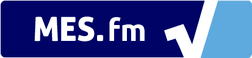We notice that you are using AdBlock. Please whitelist Percentage Calculator to help keep this site up and running! :)# Percentage Calculator

Our Percent Calculator calculates percentages based on various inputs including ratios, fractions, percentage grades, statistics and percentage increase/decrease.

The calculations and formulas (press the '?' button) are calculated automatically as you type!

Learn the basics of percentages: How to Calculate Percentages

is what percentage of  ?
Formula:  A / B * 100
What is  % of  ?
Formula:  A * B / 100
is  % of what?
Formula:  A / B * 100
Increase/Decrease from  to  ?
Formula:  (B - A) / A * 100
Percentage of the mixed fraction:
?
Formula:  (A * C + B) / C * 100
x  % ?
Formula:  A * B / 100
/  % ?
Formula:  A / (B / 100)
Increase  by  %
Formula:  A * (1 + B / 100)
Decrease  by  %
Formula:  A * ( 1 - B / 100)

## Save Time with your Keyboard

Our percentage calculator is perfect for anyone that wants to save time in calculating many different percentages as well as for anyone that is not good at math! To even save you more time we made sure that the calculations are automatically calculated as you type in the input boxes. Another way we have made it to save you time is, if you are using a computer, you can simply move from one input to the next by pressing “Tab” on your keyboard and to move to the previous input press “Shift + Tab” together on your keyboard! This makes it super easy and fast to move to each input without needing to use your mouse or cursor.

## Scientific Notation

If you are inputting numbers in the percentage calculator that result in an answer being very small or very large, the answer may appear in the format of scientific notation to fit inside the answer box. Scientific Notation is simply a number format that includes a multiplication of 10 to the power of either a negative number, for small numbers, or to the power of a positive number, for larger numbers. This method reduces the amount of digits and especially zeros needed to write in representing a number.

Some examples of scientific notation are shown below:

### Example 1: Scientific Notation for Small Numbers

0.000027 = 2.7x10-5

270000 = 2.7x105

## Formulas of calculations

The formulas of each of the calculations used in our percentage calculator can be seen by clicking the question mark, , next to each calculation. A video tutorial on using our calculator as well as a walk through of the calculations and formulas is shown here: Percentage Calculator Tutorial.